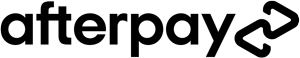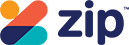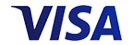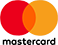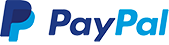Grid ListWAS \$49.95 NOW \$37.45
Increase value Decrease valueWAS \$47.95 NOW \$35.95
Increase value Decrease valueWAS \$47.95 NOW \$35.95
Increase value Decrease value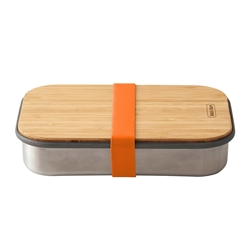WAS \$56.95 NOW \$42.70
Increase value Decrease value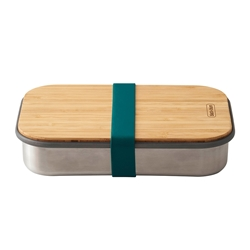WAS \$56.95 NOW \$42.70
Increase value Decrease value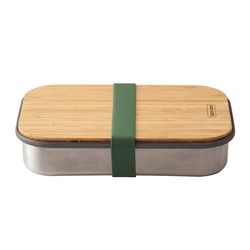WAS \$56.95 NOW \$42.70
Increase value Decrease value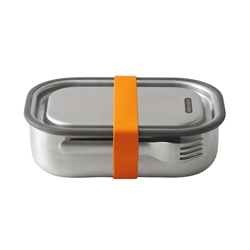WAS \$59.95 NOW \$44.95
Increase value Decrease value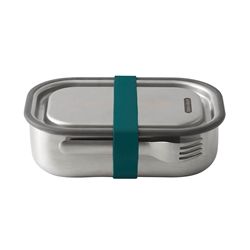WAS \$59.95 NOW \$44.95
Increase value Decrease value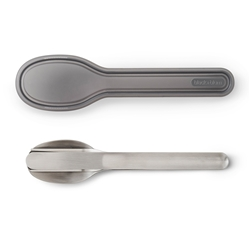WAS \$24.95 NOW \$18.70
Increase value Decrease valueWAS \$39.95 NOW \$29.95
Increase value Decrease valueWAS \$39.95 NOW \$29.95
Increase value Decrease value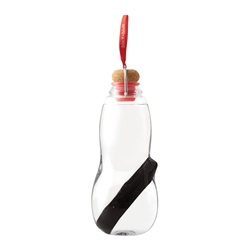WAS \$39.95 NOW \$29.95
Increase value Decrease value